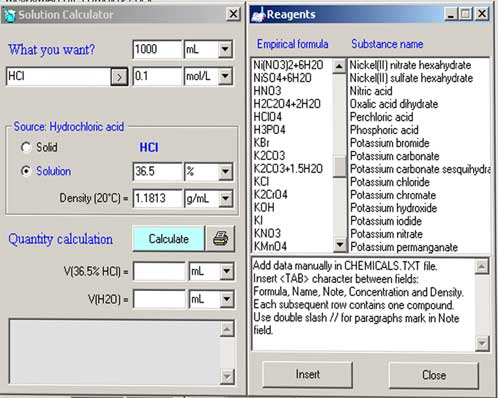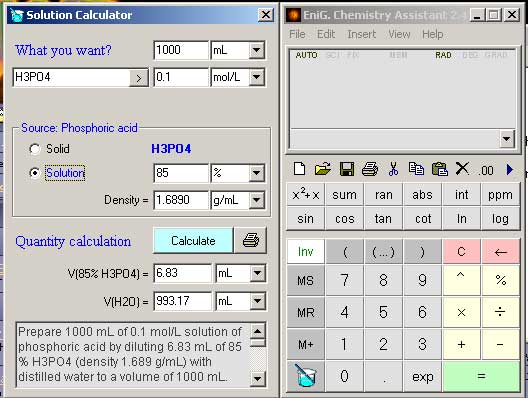###Author Topic: Calculating moles software  (Read 2079 times)

0 Members and 1 Guest are viewing this topic.

#### wareami

• Guest##### Calculating moles software
« on: January 11, 2004, 02:18:00 AM »
Five Thumbs UP!Ibee highly recommends this program...it's freeware!

EniG. Chemistry Assistant

(http://www.ktf-split.hr/~eni/toys/chemas-e.html)
Version: 2.4
Revision Date: 2002/12/19
Type of software: Freeware
Operating system: Windows 9x/NT/2000/XP

Required file: msvbvm60.dll, comdlg32.ocx
with all files: full_ca24.zip (968 kB)

without VB library: short_ca24.zip (225 kB)
Install and run.
Click on the beaker in the bottom left hand corner to bring up the leftside window to select reagents.Manually input the reagent you want or select from dropdown menu.
It took 3 minutes to figure out how to create these outputs!Prepare 1000 mL of 0.1 mol/L solution of phosphoric acid by diluting 6.74 mL of 85 % H3PO4 (density 1.71 g/mL) with distilled water to a volume of 1000 mL. The dilution is therefore made by adding 993.26 mL of water. However, the volume of a liquid obtained by mixing measured volumes of diferent solutions is not always precisely the sum of the component volumes.

NOTE:
¯¯¯¯¯¯
Ortophosphoric acid
85% H3PO4
Colorless, odorless, sparkling liquid.
Molar mass = 97.9952 g/mol
CAS No. [7664-38-2]
Boiling point 158°C
Density (85%, 20°C) 1.71 g/mL
Soluble in water
pH value (20°C): <0.5

CALCULATION:
¯¯¯¯¯¯¯¯¯¯¯¯¯
Source (B): 85 % solution of H3PO4 (density 1.71 g/mL)

The molar concentration of a solution of a chemical species A is the number of moles of that species contained in one liter of the solution (not in one liter of the solvent).

c(A) = 0.1 mol/L

Molar concentration = (Density * Weight percent  / 100) / Molecular weight

c(B) = (d(B) * w(B) / 100) / M(B)
c(B) = (1710 g/L * 85 % / 100) / 97.9952 g/mol
c(B) = 14.8323591359577 mol/L

The number of moles of solute in the diluted solution must equal the number of moles in the concentrated reagent.

V(A) * c(A) = V(B) * c(B)

V(B) = V(A) * c(A) / c(B)
V(B) = 1 L * 0.1 mol/L / 14.8323591359577 mol/L
V(B) = 6.74201582387341E-03 L
V(B) = 6.74 mL

Prepare 1000 mL of 0.1 mol/L H3PO3 by diluting 9.65 mL of 85 % H3PO3 (density 1 g/mL) with distilled water to a volume of 1000 mL. The dilution is therefore made by adding 990.35 mL of water. However, the volume of a liquid obtained by mixing measured volumes of diferent solutions is not always precisely the sum of the component volumes.

CALCULATION:
¯¯¯¯¯¯¯¯¯¯¯¯¯
Source (B): 85 % solution of H3PO3 (density 1 g/mL)

The molar concentration of a solution of a chemical species A is the number of moles of that species contained in one liter of the solution (not in one liter of the solvent).

c(A) = 0.1 mol/L

Molar concentration = (Density * Weight percent  / 100) / Molecular weight

c(B) = (d(B) * w(B) / 100) / M(B)
c(B) = (1000 g/L * 85 % / 100) / 81.9958 g/mol
c(B) = 10.3663846197 mol/L

The number of moles of solute in the diluted solution must equal the number of moles in the concentrated reagent.

V(A) * c(A) = V(B) * c(B)

V(B) = V(A) * c(A) / c(B)
V(B) = 1 L * 0.1 mol/L / 10.3663846197 mol/L
V(B) = 9.64656470588235E-03 L
V(B) = 9.65 mL

#### embezzler

• Guest##### thanks
« Reply #1 on: January 14, 2004, 10:32:00 PM »
thanks :excellant piece of software, nice find, this will cut down lab calculation time#### ClearLight

• Guest##### Wow!
« Reply #2 on: January 16, 2004, 09:09:00 PM »
Someone plz edit that txt file and put all our goodies in it for look up!!!

Rhod should put this on his site!  big score!!